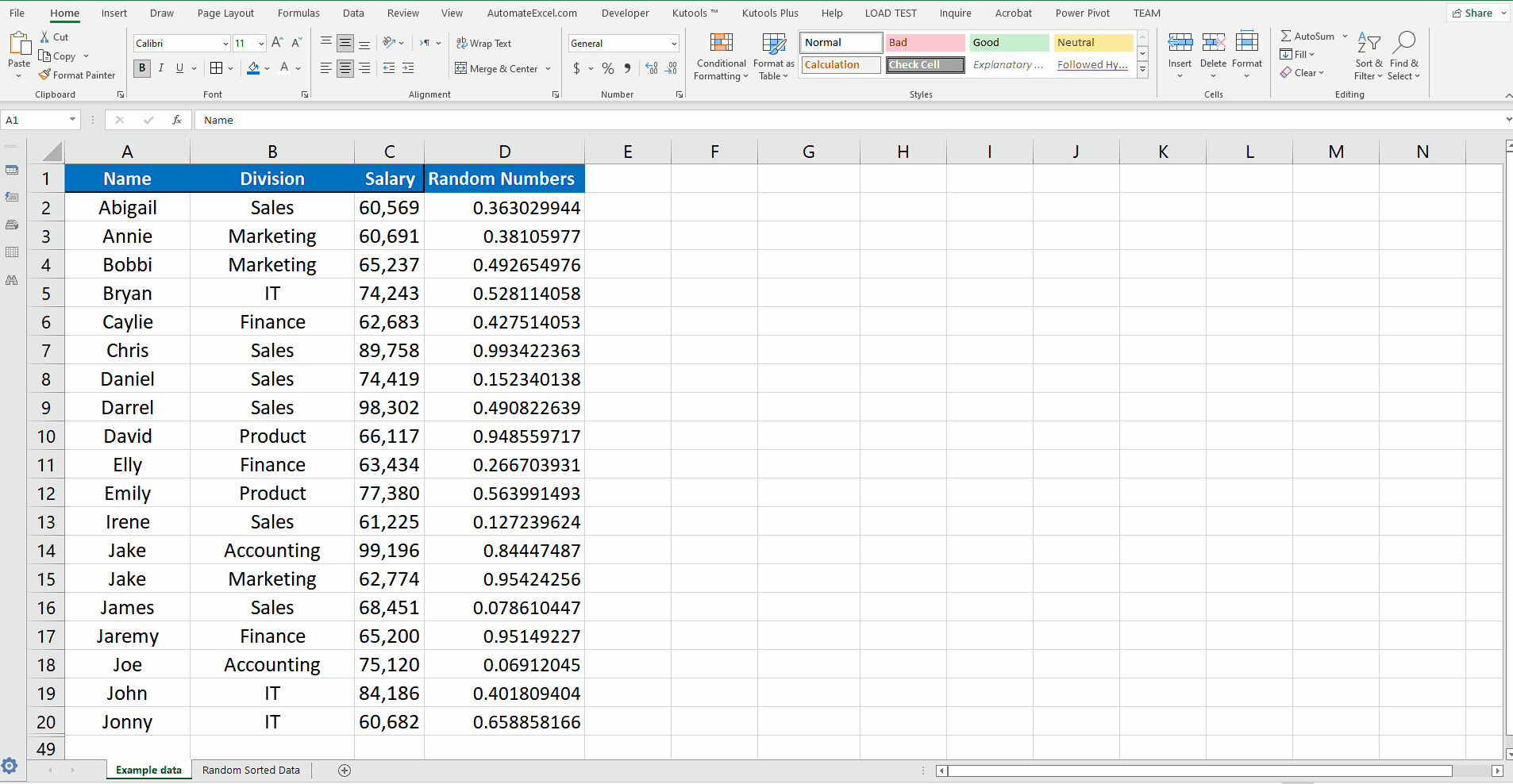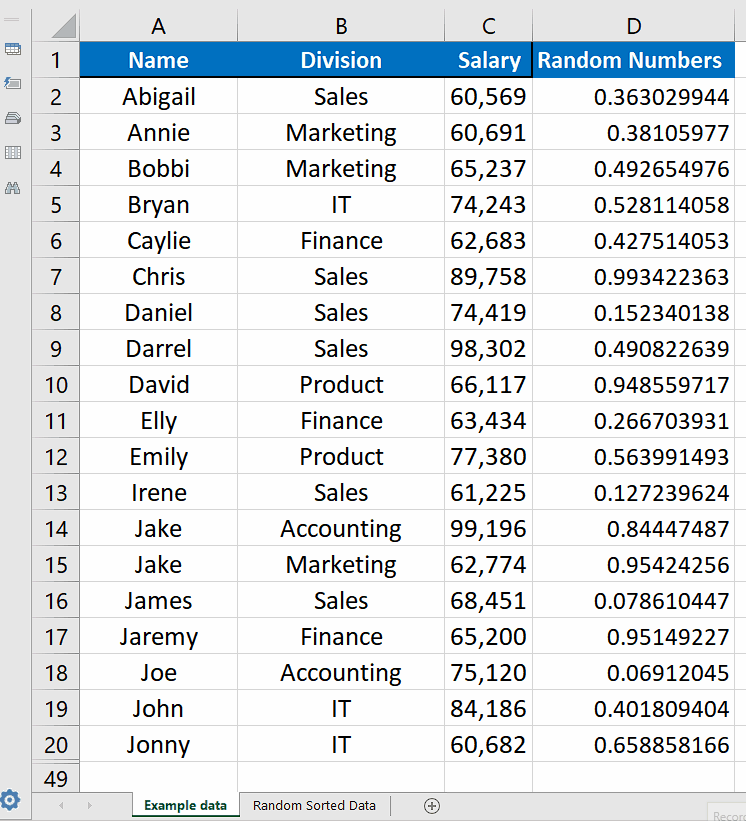# How to randomize a list in Excel

So, in today’s tutorial we will learn how to randomize a list containing text or numeric data in Excel. Let’s consider this dataset where we have some employee names, division and respective salaries. We’ll learn how to randomize the data with respect to the names of the employees.Excel is a very useful tool for analyzing data and performing various calculations with the data. It has some very powerful functions and tools to manipulate text data as well. One such tool is Sort which has the ability to sort all types of data as per requirements. However, it has its limitations and can sort the numeric data in ascending or descending orders and text data alphabetically only. This function can’t sort the list of numbers or text data randomly.

### Step 1 – Create a random number column– As excel doesn’t have a direct formula to randomly sort the data therefore, we have to do it using a workaround. We will create a helper column outside the data and name it Random Numbers. Now create random numbers in this column using a simple formula of RAND(). This will create a random number between 0 and 1, as shown above.

### Step 2 – Use Select all data including the new column– After creating the helper column let’s select all the data including the new helper column and then we’ll sort the data randomly.

### Step 3 – Use Sort and Filter and then Custom Sort to randomize list– Now go to the Editing group from the main menu and click on Sort & Filter —> Custom Sort.
– A new dialog will be opened and in that dialog under Column —> Sort by, choose the column Random Numbers and press OK button. This will sort the data based on the random numbers which were generated randomly. So the data will be sorted randomly. The good thing about this method is that the row data will remain intact i.e. the respective rows retain their data as it was in original data.
– If you are happy with one iteration then you can remove the helper column or if you want to try another iteration then you can repeat the process above to create another list.